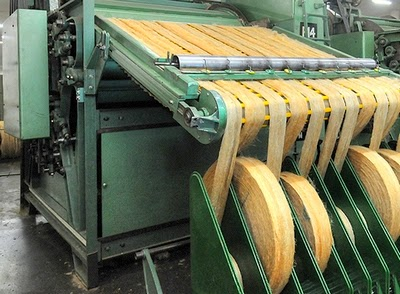# Textile Calculation

Problems and solutions of textile

## Jute Carding Calculation Formula

Some Important Jute Carding Calculation FormulasMd. Abu Sayed
Founder of Textile Apex
Pabna Textile engineering college, Pabna, Bangladesh
Cell : +8801745214773

Jute Carding:
In jute spinning, carding is the major area for jute yarn production process. In jute carding machine the primary function of jute carding is done by the action of worker and cylinder and the cleaning and the cleaning actions is done between worker and stripper. For jute yarn production, some calculation formulas of jute carding are given below.Jute Carding
Some important formulas for jute carding –
Surface speed (SS) of cylinder roller – SS of worker roller
1. Carding efficiency = ………………………………………………………………
Surface speed (SS) of cylinder

SS of cylinder
2. Carding Ratio = ……………………………
SS of worker

Dollop wt.
3. Wt. of feed sliver/100 yd = ……………………… X 100%
Clock length

Doubling
4. Wt. of delivery/100 yd = wt. of feed sliver/100yd X …………………..
Draft

Dollop wt.                                Doubling
= …………………… X 100 X …………………..
Clock length                                Draft

SS of delivery roller
5. Mechanical Draft = ……………………………
SS of feed roller

Calculated feed sliver wt. /100 yd
= ……………………………………………………………..
Calculated delivered sliver wt. /100 yd

Wt. /100 yd of feed material
6. Actual draft = ……………………………………….. X Doubling X Waste%
Wt. /100 yd of delivered material

= Mechanical draft X Waste%

7. Draft constant = DCP X Draft

8. SS of feed roller = π X Dia in inch X Speed

Length of feed material
= ……………………………………….
Doubling

SS of delivery roller
= ………………………………….
Draft

9. SS of delivery roller = π  X  Dia in inch X Speed

= SS of delivery roller X Lead%

10. Finisher card sliver wt. /100 yd

B/C sliver wt. /100 yd
= ………………………………………. X Doubling X Waste%
F/C draft

Dollop wt.                    B/C doubling          F/C doubling
= …………………… X 100 X ………………… X ………………… X Waste%
Clock length                   B/C draft                  F/C draft

11. Production /hr = SS of delivery roller in inch /min X 60/36 X wt. of sliver/yd X Eff% X Waste%

Dollop wt.
= SS of feed roller in inch/min X 60/36 X ……………………. X Eff% X Waste%
Clock length

Some Problems and Solutions:

1. The surface speed of cylinder is 2400 feet/min. If the carding efficiency 90%, then what will be the SS of worker roller.

Solution:
SS of cylinder – SS of worker
Carding efficiency = …………………………………………………… X 100
SS of cylinder

=> 90 = (2400 – x) / 2400 X 100 (Let, SS of worker roller be x)
=> 90 X 2400 = 240000 – 100x
=> 100x = 240000 – 216000
=> X = 2400/100 = 240 ft/min (ANS)
2. Find out the wt/100yd of BC sliver when,
• Dollop wt. = 30 lb
• Clock length = 12.23
• Draft = 11.23
• Evaporation and short fibre loss = 4%
Solution:

We know, Breaker card sliver wt. /100yd = (Dollop wt.) / (Clock length) X 100 X Doubling / Draft X Waste%

= 30/12.9 X 100 X 1/11.23 X (100 – 4)/100

= 19.88 lb/100yd. (ANS)

3. If B/C draft is 12, dollop wt. is 38 lb and Clock length (CL) is 14.4 yd, Then find –
• Feed sliver wt./100yd,
• Delivered sliver wt./100yd.
Solution:

Feed sliver wt./100 yd = DW/CL X 100
= 38/14.4 X 100
= 263.89 lb.

Delivered sliver wt./100yd = 263.89/Draft
= 263.89/12
= 21.99 lb. (ANS)

4. A B/C is working with 14.4 yd CL having FR speed of 6.94 rpm and dia 9.75 inch. What will be the time reqd to feed one dollop of 36 lb?

Solution:
Given,
• CL = 14.4 yd
• FR speed = 6.94 rpm
• FR dia = 9.75 inch
• Dollop wt. = 36 lb
• Time to finish one dollop =?
Distance traversed by the feed roller in one min = πdn = π X 9.75 X 6.94 inch/min

= 3.14 X 9.75/36 X 6.94 yd/min
= 5.90 yd/min.

Time required to feed one dollop = (CL in yd)/SS of FR in yd
= 14.4/5.9 min = 2.44 min (ANS)

5. A B/C is running with a CL 12.9 yd and DW 30 lb. having SS of feed roller 18 feet/min. If the efficiency of B/C is 80% and evaporation loss is 4%, then what will be the production/shift in metric ton? [1 MT = 2204 lb]

Solution:
Given,
• CL = 12.9 yd
• DW = 30 lb
• SS of feed roller = 18 feet/min
• Evaporation loss = 4%
• Efficiency =80%
• Production/shift =?
We know,

Production/shift = SS of feed roller in inch/min X 60 X DW/Cl X Eff% X Waste%
= 18/3 X 30/12.9 X 80/100 X (100 – 4)/100 X 60 X 8 X 1/2204
= 2.33 (ANS)